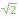# SQRT2

 Block Group: Constants Icon:The SQRT2 is a Number block. When the SQRT2 block is created, the SQRT2 block holds the mathematical constant for the square root of two.

For information on using dataflow blocks, see Dataflow.

## Input/Output Property

The following property of the SQRT2 block can take input and give output.

• value (number)

value sets and returns the number held by this SQRT2 block. When a SQRT2 block is created, the value property holds the mathematical constant for the square root of two.

## Example

The following image demonstrates an example of the SQRT2 block.